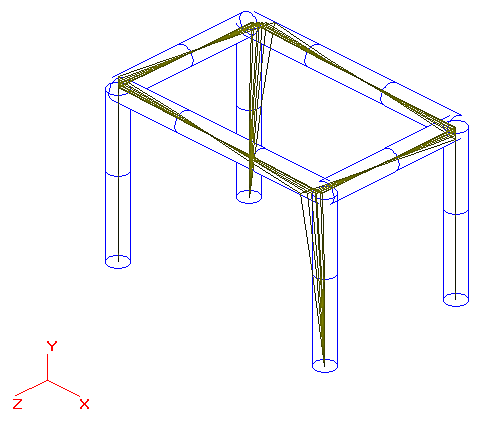# Harmonic Analysis of this System - CAESAR II - Reference Data

## CAESAR II Applications Guide (2019 Service Pack 1)

PPMProduct
CAESAR II
PPMCategory_custom
Reference Data
Version_CAESAR
11.0 (2019)

In this example, a 120 Hz electric motor sits on the piping structure and acts:

FX @ 8 = (-95 cos w t ) lb.

FX @ 13 = (95 cos w t ) lb.

The harmonic analysis can determine the largest stress in the small piping structure subject to these dynamic loads.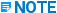The 120 Hz vibration falls between the structural resonant frequencies 115 Hz and 137 Hz. The torsional mode is most likely excited because the sign difference on the forces promotes a twisting of the structure. The model has already been built, so dynamic input only requires a slight modification. There is only a single harmonic frequency of excitation to investigate.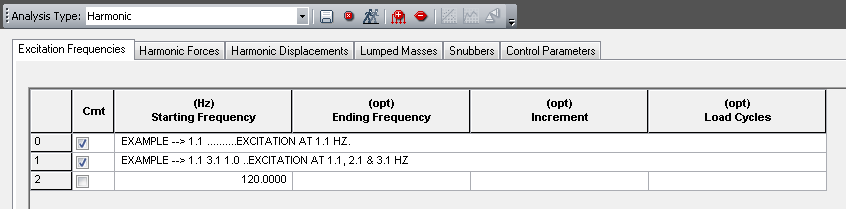You enter harmonic loads next. The software prompts you for harmonic forces first and then prompts you for direction. In the example piping system, harmonic forces act at nodes 8 and 13. The forces act in the X-direction with an opposite sign and with a magnitude of 95 pounds. The force acting at node 8 can be plotted as a function of time as shown in the figure below.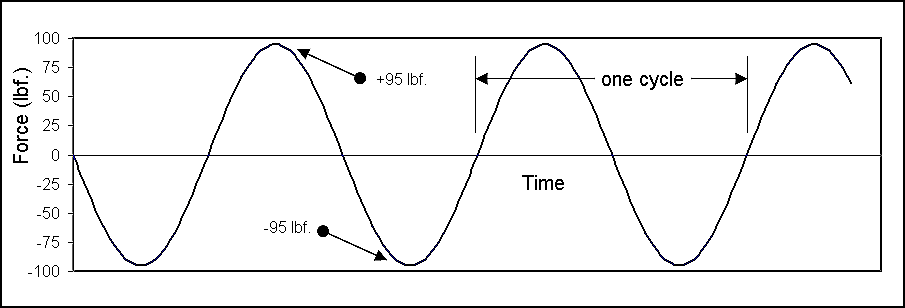In the example problem, there are 120 cycles per second.

The following figure shows the harmonic force data input. If necessary, harmonic displacements can exist in the same problem with harmonic forces. This example has only harmonic forces.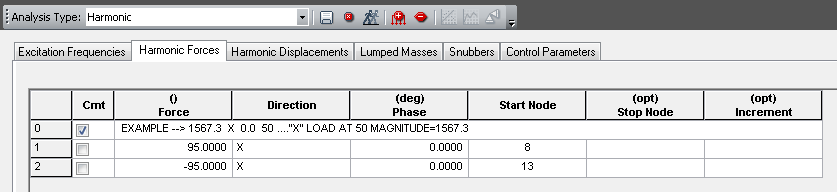The same force effect can be achieved by entering +95.0 pounds at each node and entering a phase angle of 180.0 degrees at node 13.

Calculations for the example problem take less than 30 seconds to complete. You can view the structure in animated motion or view standard displaced shape plots in the DynPlot window using the Display Graphical Results option (as shown below). Additionally, harmonic results, restraint loads, forces, and stresses can be calculated for the maximum displacements due to the harmonic loads.